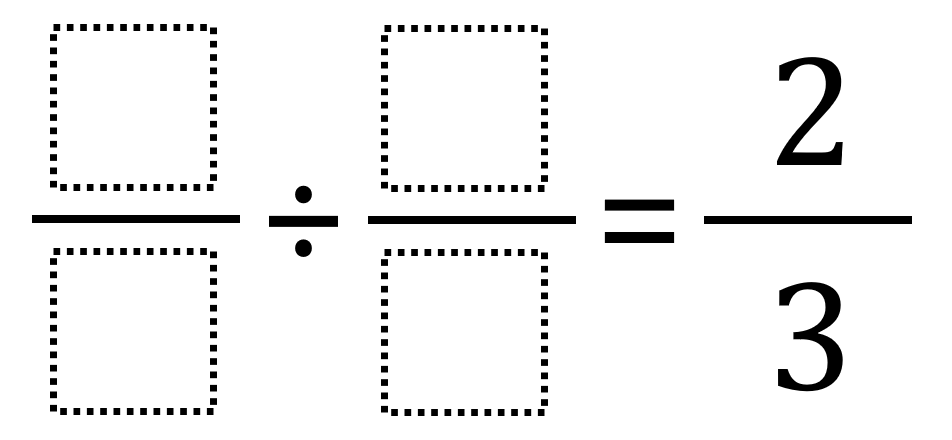# Dividing Fractions To Make 2/3

Directions: Using the digits 1 to 9 at most one time each, place a digit in each box to make two different pairs of fractions that have a quotient of 2/3.### Hint

How can you make multiple fractions with equivalent values? How might you think of this problem as either division or multiplication?

There are many potential answers including 4/2 ÷ 3/1

Source: Robert Kaplinsky in Open Middle Math

## Greatest Common Factor

Directions: Using the digits 0 to 9 at most one time each, place a digit …

### One comment

1.If I understood the problem correctly, I found 3/2 ÷ 9/4 and 2/6 ÷ 4/8